The STATESPACE Procedure

Automatic State Space Model Selection

Subsections:

The STATESPACE procedure is designed to automatically select the best state space model for forecasting the series. You can specify your own model if you want, and you can use the output from PROC STATESPACE to help you identify a state space model. However, the easiest way to use PROC STATESPACE is to let it choose the model.

Stationarity and Differencing

Although PROC STATESPACE selects the state space model automatically, it does assume that the input series are stationary. If the series are nonstationary, then the process might fail. Therefore the first step is to examine your data and test to see if differencing is required. (See the section Stationarity and Differencing for further discussion of this issue.)

The series shown in Figure 28.1 are nonstationary. In order to forecast X and Y with a state space model, you must difference them (or use some other detrending method). If you fail to difference when needed and try to use PROC STATESPACE with nonstationary data, an inappropriate state space model might be selected, and the model estimation might fail to converge.

The following statements identify and fit a state space model for the first differences of X and Y, and forecast X and Y 10 periods ahead:

proc statespace data=in out=out lead=10;
var x(1) y(1);
id t;
run;


The DATA= option specifies the input data set and the OUT= option specifies the output data set for the forecasts. The LEAD= option specifies forecasting 10 observations past the end of the input data. The VAR statement specifies the variables to forecast and specifies differencing. The notation X(1) Y(1) specifies that the state space model analyzes the first differences of X and Y.

Descriptive Statistics and Preliminary Autoregressions

The first page of the printed output produced by the preceding statements is shown in Figure 28.2.

Figure 28.2: Descriptive Statistics and VAR Order Selection

The STATESPACE Procedure

 Number of Observations 200

Variable Mean Standard
Error

x 0.144316 1.233457 Has been differenced. With period(s) = 1.
y 0.164871 1.304358 Has been differenced. With period(s) = 1.

The STATESPACE Procedure

Information Criterion for Autoregressive Models
Lag=0 Lag=1 Lag=2 Lag=3 Lag=4 Lag=5 Lag=6 Lag=7 Lag=8 Lag=9 Lag=10
149.697 8.387786 5.517099 12.05986 15.36952 21.79538 24.00638 29.88874 33.55708 41.17606 47.70222

Schematic Representation of Correlations
Name/Lag 0 1 2 3 4 5 6 7 8 9 10
x ++ ++ ++ ++ ++ ++ +. .. +. +. ..
y ++ ++ ++ ++ ++ +. +. +. +. .. ..

Descriptive statistics are printed first, giving the number of nonmissing observations after differencing and the sample means and standard deviations of the differenced series. The sample means are subtracted before the series are modeled (unless the NOCENTER option is specified), and the sample means are added back when the forecasts are produced.

Let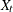and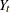be the observed values of X and Y, and let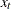and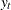be the values of X and Y after differencing and subtracting the mean difference. The series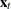modeled by the STATEPSPACE procedure is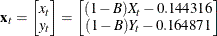where B represents the backshift operator.

After the descriptive statistics, PROC STATESPACE prints the Akaike information criterion (AIC) values for the autoregressive models fit to the series. The smallest AIC value, in this case 5.517 at lag 2, determines the number of autocovariance matrices analyzed in the canonical correlation phase.

A schematic representation of the autocorrelations is printed next. This indicates which elements of the autocorrelation matrices at different lags are significantly greater than or less than 0.

The second page of the STATESPACE printed output is shown in Figure 28.3.

Figure 28.3: Partial Autocorrelations and VAR Model

Schematic Representation of Partial
Autocorrelations
Name/Lag 1 2 3 4 5 6 7 8 9 10
x ++ +. .. .. .. .. .. .. .. ..
y ++ .. .. .. .. .. .. .. .. ..

Yule-Walker Estimates for Minimum AIC
Lag=1 Lag=2
x y x y
x 0.257438 0.202237 0.170812 0.133554
y 0.292177 0.469297 -0.00537 -0.00048

Figure 28.3 shows a schematic representation of the partial autocorrelations, similar to the autocorrelations shown in Figure 28.2. The selection of a second order autoregressive model by the AIC statistic looks reasonable in this case because the partial autocorrelations for lags greater than 2 are not significant.

Next, the Yule-Walker estimates for the selected autoregressive model are printed. This output shows the coefficient matrices of the vector autoregressive model at each lag.

Selected State Space Model Form and Preliminary Estimates

After the autoregressive order selection process has determined the number of lags to consider, the canonical correlation analysis phase selects the state vector. By default, output for this process is not printed. You can use the CANCORR option to print details of the canonical correlation analysis. See the section Canonical Correlation Analysis Options for an explanation of this process.

After the state vector is selected, the state space model is estimated by approximate maximum likelihood. Information from the canonical correlation analysis and from the preliminary autoregression is used to form preliminary estimates of the state space model parameters. These preliminary estimates are used as starting values for the iterative estimation process.

The form of the state vector and the preliminary estimates are printed next, as shown in Figure 28.4.

Figure 28.4: Preliminary Estimates of State Space Model

The STATESPACE Procedure
Selected Statespace Form and Preliminary Estimates

State Vector
x(T;T) y(T;T) x(T+1;T)

Estimate of Transition Matrix
0 0 1
0.291536 0.468762 -0.00411
0.24869 0.24484 0.204257

Input Matrix for Innovation
1 0
0 1
0.257438 0.202237

Variance Matrix for Innovation
0.945196 0.100786
0.100786 1.014703

Figure 28.4 first prints the state vector as   X[T;T]   Y[T;T]   X[T+1;T]. This notation indicates that the state vector is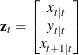The notation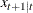indicates the conditional expectation or prediction of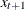based on the information available at time t, and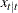and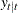areand, respectively.

The remainder of Figure 28.4 shows the preliminary estimates of the transition matrix
, the input matrix, and the covariance matrix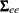.

Estimated State Space Model

The next page of the STATESPACE output prints the final estimates of the fitted model, as shown in Figure 28.5. This output has the same form as in Figure 28.4, but it shows the maximum likelihood estimates instead of the preliminary estimates.

Figure 28.5: Fitted State Space Model

The STATESPACE Procedure
Selected Statespace Form and Fitted Model

State Vector
x(T;T) y(T;T) x(T+1;T)

Estimate of Transition Matrix
0 0 1
0.297273 0.47376 -0.01998
0.2301 0.228425 0.256031

Input Matrix for Innovation
1 0
0 1
0.257284 0.202273

Variance Matrix for Innovation
0.945188 0.100752
0.100752 1.014712

The estimated state space model shown in Figure 28.5 is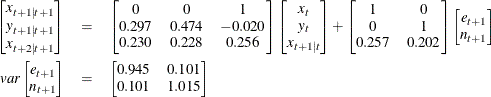The next page of the STATESPACE output lists the estimates of the free parameters in theandmatrices with standard errors and t statistics, as shown in Figure 28.6.

Figure 28.6: Final Parameter Estimates

Parameter Estimates
Parameter Estimate Standard
Error
t Value
F(2,1) 0.297273 0.129995 2.29
F(2,2) 0.473760 0.115688 4.10
F(2,3) -0.01998 0.313025 -0.06
F(3,1) 0.230100 0.126226 1.82
F(3,2) 0.228425 0.112978 2.02
F(3,3) 0.256031 0.305256 0.84
G(3,1) 0.257284 0.071060 3.62
G(3,2) 0.202273 0.068593 2.95

Convergence Failures

The maximum likelihood estimates are computed by an iterative nonlinear maximization algorithm, which might not converge. If the estimates fail to converge, warning messages are printed in the output.

If you encounter convergence problems, you should recheck the stationarity of the data and ensure that the specified differencing orders are correct. Attempting to fit state space models to nonstationary data is a common cause of convergence failure. You can also use the MAXIT= option to increase the number of iterations allowed, or experiment with the convergence tolerance options DETTOL= and PARMTOL=.

Forecast Data Set

The following statements print the output data set. The WHERE statement excludes the first 190 observations from the output, so that only the forecasts and the last 10 actual observations are printed.

proc print data=out;
id t;
where t > 190;
run;


The PROC PRINT output is shown in Figure 28.7.

Figure 28.7: OUT= Data Set Produced by PROC STATESPACE

t x FOR1 RES1 STD1 y FOR2 RES2 STD2
191 34.8159 33.6299 1.18600 0.97221 58.7189 57.9916 0.72728 1.00733
192 35.0656 35.6598 -0.59419 0.97221 58.5440 59.7718 -1.22780 1.00733
193 34.7034 35.5530 -0.84962 0.97221 59.0476 58.5723 0.47522 1.00733
194 34.6626 34.7597 -0.09707 0.97221 59.7774 59.2241 0.55330 1.00733
195 34.4055 34.8322 -0.42664 0.97221 60.5118 60.1544 0.35738 1.00733
196 33.8210 34.6053 -0.78434 0.97221 59.8750 60.8260 -0.95102 1.00733
197 34.0164 33.6230 0.39333 0.97221 58.4698 59.4502 -0.98046 1.00733
198 35.3819 33.6251 1.75684 0.97221 60.6782 57.9167 2.76150 1.00733
199 36.2954 36.0528 0.24256 0.97221 60.9692 62.1637 -1.19450 1.00733
200 37.8945 37.1431 0.75142 0.97221 60.8586 61.4085 -0.54984 1.00733
201 . 38.5068 . 0.97221 . 61.3161 . 1.00733
202 . 39.0428 . 1.59125 . 61.7509 . 1.83678
203 . 39.4619 . 2.28028 . 62.1546 . 2.62366
204 . 39.8284 . 2.97824 . 62.5099 . 3.38839
205 . 40.1474 . 3.67689 . 62.8275 . 4.12805
206 . 40.4310 . 4.36299 . 63.1139 . 4.84149
207 . 40.6861 . 5.03040 . 63.3755 . 5.52744
208 . 40.9185 . 5.67548 . 63.6174 . 6.18564
209 . 41.1330 . 6.29673 . 63.8435 . 6.81655
210 . 41.3332 . 6.89383 . 64.0572 . 7.42114

The OUT= data set produced by PROC STATESPACE contains the VAR and ID statement variables. In addition, for each VAR statement variable, the OUT= data set contains the variables FORi, RESi, and STDi. These variables contain the predicted values, residuals, and forecast standard errors for the ith variable in the VAR statement list. In this case, X is listed first in the VAR statement, so FOR1 contains the forecasts of X, while FOR2 contains the forecasts of Y.

The following statements plot the forecasts and actuals for the series.

proc sgplot data=out noautolegend;
where t > 150;
series x=t y=for1 / markers
markerattrs=(symbol=circle color=blue)
lineattrs=(pattern=solid color=blue);
series x=t y=for2 / markers
markerattrs=(symbol=circle color=blue)
lineattrs=(pattern=solid color=blue);
series x=t y=x / markers
markerattrs=(symbol=circle color=red)
lineattrs=(pattern=solid color=red);
series x=t y=y / markers
markerattrs=(symbol=circle color=red)
lineattrs=(pattern=solid color=red);
refline 200.5 / axis=x;
run;


The forecast plot is shown in Figure 28.8. The last 50 observations are also plotted to provide context, and a reference line is drawn between the historical and forecast periods.

Figure 28.8: Plot of Forecasts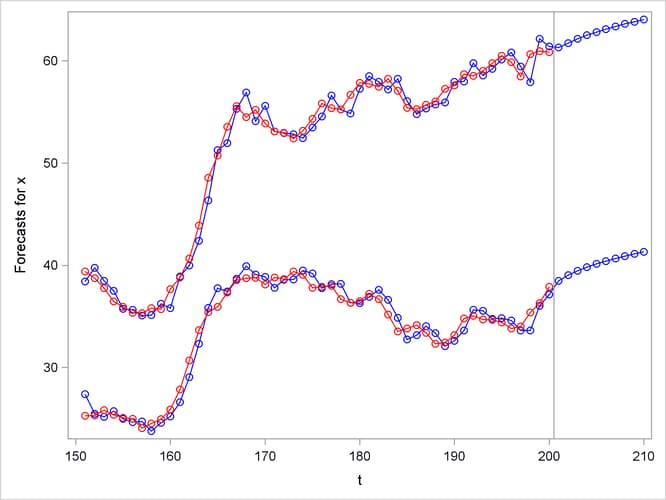Controlling Printed Output

By default, the STATESPACE procedure produces a large amount of printed output. The NOPRINT option suppresses all printed output. You can suppress the printed output for the autoregressive model selection process with the PRINTOUT=NONE option. The descriptive statistics and state space model estimation output are still printed when PRINTOUT=NONE is specified. You can produce more detailed output with the PRINTOUT=LONG option and by specifying the printing control options CANCORR, COVB, and PRINT.